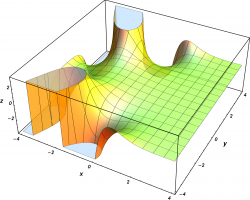# Analysis and Partial Differential EquationsAnalysis includes operator theory and C*-algebras – the study of infinite dimensional systems of equations, Fourier analysis – the study of infinite dimensional trigonometric systems, complex analysis – the study of functions of a complex variable, partial differential equations and dynamical systems – the equations which model how physical quantities change with time or space, and research in theoretical and mathematical physics.

### Our areas of expertise

Our analysis group has several broad research interests that include C*-algebras, complex analysis (one and several variables), Fourier analysis, partial differential equations and dynamical systems.

C*-algebras is the study of algebraic systems of linear operators on Hilbert space, which grew out of quantum mechanics in the first half of the 20th century, and has been applied in many ways, including quantum field theory, knot theory, number theory, and tilings.

Complex and Fourier analysis examine the properties that distinguish functions which can be expressed as power series and/or trigonometric expansions. Research in these subjects has applications to signal and image analysis and many other areas of science and engineering.

Partial differential equations are mathematical expressions of physical laws with applications to many areas, including mathematical physics, atmospheric sciences, and mathematical biology. Dynamical systems are characterized by a time evolution operator and my arise from  partial differential equations, differential-delay, integral and difference equations (notably in mathematical biology).

Methods of analysis are applied to fundamental problems in theoretical and mathematical physics, including classical and quantum electrodynamics, relativistic quantum mechanics, quantum optics and nonlinear waves.